# Solved Problems On Zener Diode

Q1. For the circuit shown in Fig.1 (i), find : (i) the output voltage (ii) the voltage drop across series resistance (iii) the current through zener diode.Fig.1 (i)

Solution :

If you remove the zener diode in Fig. 1, the voltage V across the open-circuit is given by :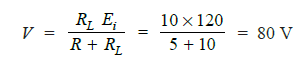Since voltage across zener diode is greater than VZ (= 50 V), the zener is in the “on” state. It can,
therefore, be represented by a battery of 50 V as shown in Fig. 1 (ii).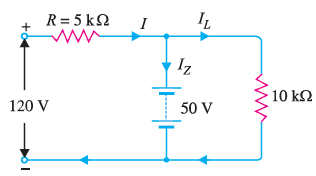Fig. 1(ii)

(i) Referring to Fig. 1 (ii),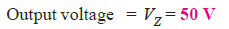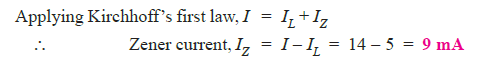Q2. For the circuit shown in Fig. 2 (i),find the maximum and minimum values of zener diode current.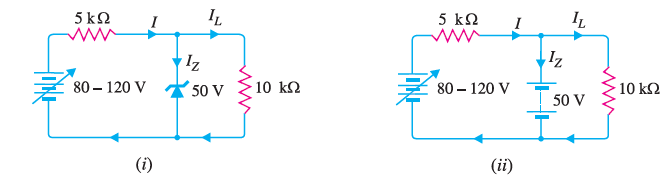Fig.2

Solution :

The first step is to determine the state of the zener diode. It is easy to see that for the given range of voltages (80 − 120 V), the voltage across the zener is greater than VZ (= 50 V). Hence the zener diode will be in the “on” state for this range of applied voltages. Consequently, it can be replaced by a battery of 50 V as shown in Fig. 2(ii).

Maximum zener current:  The zener will conduct maximum current when the input voltage is maximum i.e. 120 V. Under such conditions :Minimum Zener current: The zener will conduct minimum current when the input voltage is
minimum i.e. 80 V. Under such conditions, we have,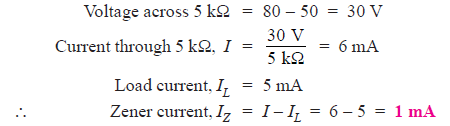Q3. A 7.2 V zener is used in the circuit shown in Fig. 3 and the load current is to vary from 12 to 100 mA. Find the value of series resistance R to maintain a voltage of 7.2 V across the load. The input voltage is constant at 12V and the minimum zener current is 10 mA.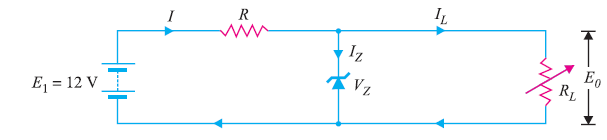Fig. 3

Solution :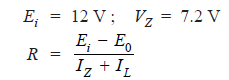The voltage across R is to remain constant at 12 − 7.2 = 4.8 V as the load current changes from 12 to 100 mA. The minimum zener current will occur when the load current is maximum.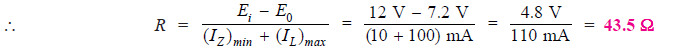If R = 43.5 Ω is inserted in the circuit, the output voltage will remain constant over the regulating range. As the load current IL decreases, the zener current IZ will increase to such a value that IZ + IL = 110 mA.

Note that if load resistance is open-circuited, then IL = 0 and zener current becomes 110 mA.

Q4. The zener diode shown in Fig. 4 has VZ = 18 V. The voltage across the load stays at 18 V as long as IZ is maintained between 200 mA and 2 A. Find the value of series  resistance R so that E0 remains 18 V while input voltage Ei is free to vary between 22 V to 28V.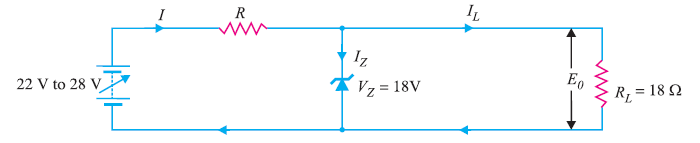Fig. 4

Solution :

The zener current will be minimum (i.e. 200 mA) when the input voltage is minimum (i.e. 22 V). The load current stays at constant value IL = VZ / RL = 18 V/18 Ω = 1 A = 1000 mA.Q5. A 10-V zener diode is used to regulate the voltage across a variable load resistor [See fig.5]. The input voltage varies between 13 V and 16 V and the load current varies between 10 mA and 85 mA. The minimum zener current is 15 mA. Calculate the value of series resistance R.

Solution :

The zener will conduct minimum current (i.e. 15 mA) when input voltage is minimum (i.e. 13 V).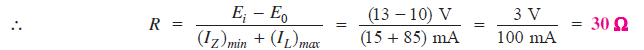Q6. The circuit of Fig. 6 uses two zener diodes, each rated at 15 V, 200 mA. If the circuit is connected to a 45-volt unregulated supply, determine :(i) The regulated output voltage (ii) The value of series resistance R.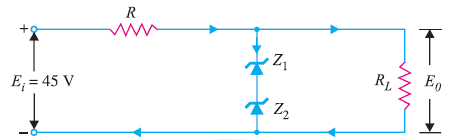Fig. 6

Solution :

When the desired regulated output voltage is higher than the rated voltage of the zener, two or more zeners are connected in series as shown in Fig. 6. However, in such circuits, care must be taken to select those zeners that have the same current rating.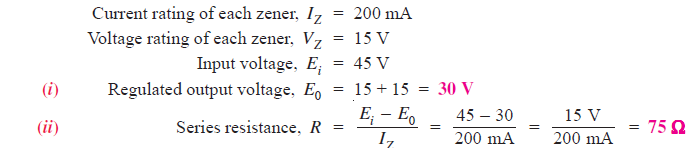Q7. What value of series resistance is required when three 10-watt, 10-volt, 1000 mA zener diodes are connected in series to obtain a 30-volt regulated output from a 45 volt d.c. power source ?

Solution :

Fig. 7 shows the desired circuit. The worst case is at no load because then zeners carry the maximum current.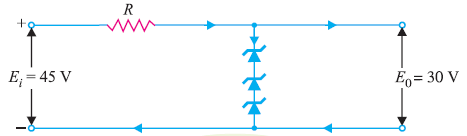Fig. 7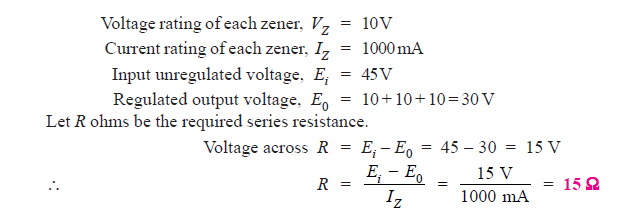Q8. Over what range of input voltage will the zener circuit shown in Fig. 8 maintain 30 V across 2000 Ω load, assuming that series resistance R = 200 Ω and zener current
rating is 25 mA ?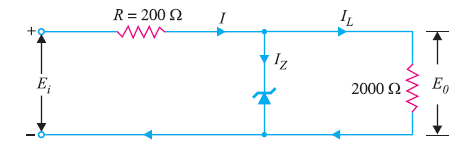Fig. 8

Solution :

The minimum input voltage required will be when IZ = 0. Under this condition,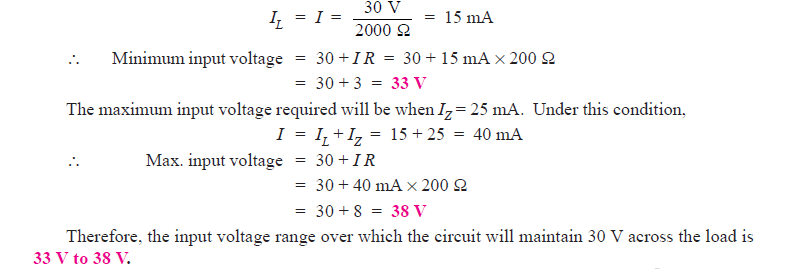Q9. In the circuit shown in Fig.9,the voltage across the load is to be maintained at 12 V as load current varies from 0 to 200 mA. Design the regulator. Also find the  maximum wattage rating of zener diode.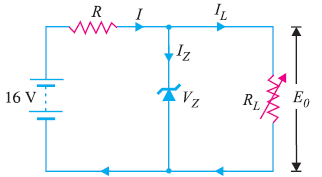Fig. 9

Solution :

By designing the regulator here means to find the values of VZ and R. Since the load voltage is to be maintained at 12 V, we will use a zener diode of zener voltage 12 V i.e.,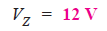The voltage across R is to remain constant at 16 − 12 = 4 V as the load current changes from 0 to 200 mA. The minimum zener current will occur when the load current is maximum.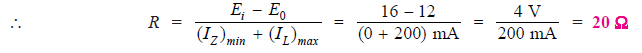Maximum power rating of zener is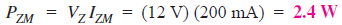Q10. Fig. 10 shows the basic zener diode circuits. What will be the circuit behaviour if the zener is (i) working properly (ii) shorted (iii) open-circuited?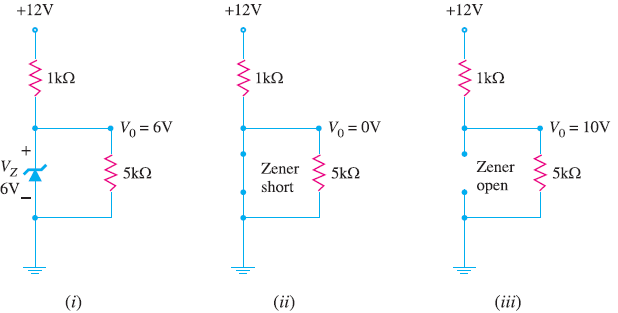Fig. 10

Solution :

Zener diodes cannot be tested individually with a multimeter. It is because multimeters usually do not have enough input voltage to put the zener into breakdown region.

(i) If the zener diode is working properly, the voltage V0 across the load (= 5 kΩ) will be nearly 6V [See Fig. 10 (i)].

(ii) If the zener diode is short [See Fig. 10 (ii)], you will measure V0 as 0V. The same problem could also be caused by a shorted load resistor (= 5kΩ) or an opened source resistor (= 1 kΩ). The only way to tell which device has failed is to remove the resistors and check them with an ohmmeter. If the resistors are good, then zener diode is bad.

(iii) If the zener diode is open-circuited, the voltage V0 across the load (= 5 kΩ) will be 10V.

Q11. Fig. 11 shows regulated power supply using a zener diode. What will be the circuit behaviour if (i) filter capacitor shorts (ii) filter capacitor opens?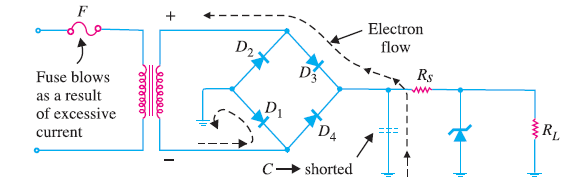Fig. 11

Solution :

The common faults in a zener voltage regulator are shorted filter capacitor or opened filter capacitor.

(i) When filter capacitor shorts :

When the filter capacitor shorts, the primary fuse will blow. The reason for this is illustrated in Fig 11. When the filter capacitor shorts, it shorts out the load resistance RL. This has the same effect as wiring the two sides of the bridge together (See Fig. 11).

If you trace from the high side of the bridge to the low side, you will see that the only resistance across the secondary of the transformer is the forward resistance of the two ON diodes. This effectively shorts out the transformer secondary. The result is that excessive current flows in the secondary and hence in the primary. Consequently, the primary fuse will blow.

(ii) When filter capacitor opens:

When the filter capacitor opens, it will cause the ripple in the power supply output to increase drastically. At the same time, the d.c. output voltage will show a significant drop. Since an open filter capacitor is the only fault that will cause both of these symptoms, no further testing is necessary. If both symptoms appear, replace the filter capacitor.